## Friday, May 27, 2016

### New options for user control of plotting function `dotTree`

I just added a couple of features to the phytools function `dotTree`. These come in the form of the two optional arguments `x.space` and `leg.space`. These arguments allow, respectively, manual control of the horizontal spacing of the columns in a grid of circles or dots in a visualization of multiple traits. Presently by default these circles have a fixed spacing, which can be too wide when trait values for a relatively small number of traits and a relatively large number of taxa are viewed.

For instance, let's start with a simulated tree & dataset with 50 taxa and 20 character traits:

``````library(phytools)
tree
``````
``````##
## Phylogenetic tree with 50 tips and 49 internal nodes.
##
## Tip labels:
##  t17, t33, t39, t15, t10, t21, ...
##
## Rooted; includes branch lengths.
``````
``````dim(X)
``````
``````##  50 20
``````
``````dotTree(tree,X,fsize=0.7)
``````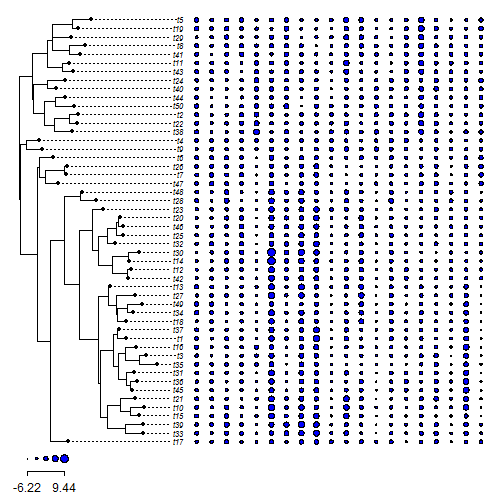This looks nice (at least in my unbiased opinion); however if we only plot two or three traits, by contrast, the columns seem over-spaced:

``````dotTree(tree,X[,1:3],fsize=0.7)
``````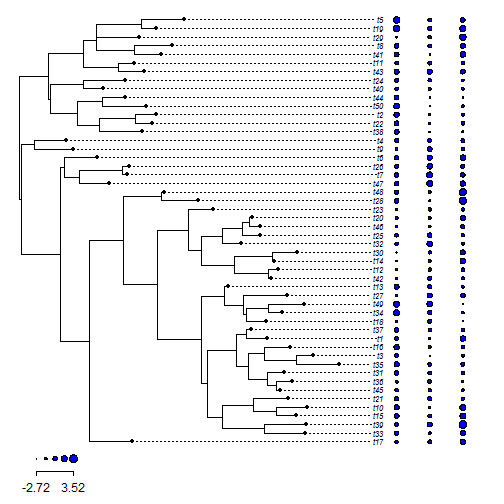The new argument, `x.space`, allows us to change the interval over which the columns are spaced:

``````dotTree(tree,X[,1:3],fsize=0.7,x.space=0.05)
``````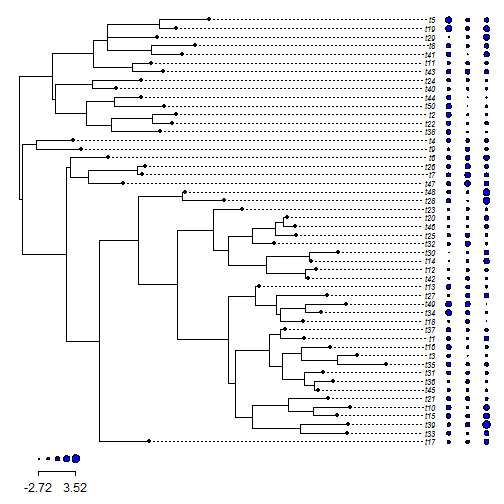The second new optional argument is `leg.space`, and this argument allows us to change the spacing on the legend. (I also changed the default value for this setting.)

``````dotTree(tree,X,fsize=0.7,leg.space=1,length=10)
``````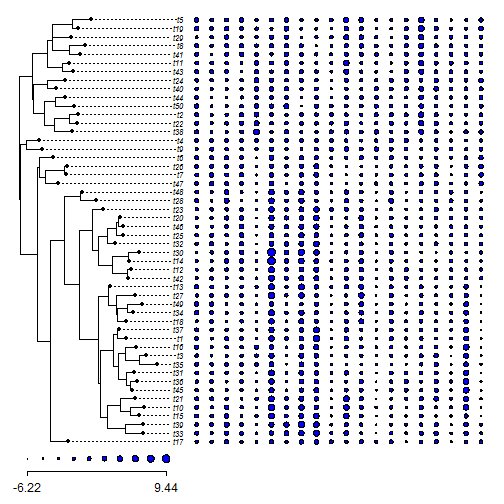(The argument `length` set the number of dots in the legend.)

Note that the argument `x.space` should also work for `data.type="discrete"` (the argument `leg.space` doesn't apply), e.g.:

``````x
``````
``````## t17 t33 t39 t15 t10 t21 t45 t36 t31 t35  t3 t16  t1 t37 t18 t34 t49 t27
## "b" "b" "b" "a" "a" "a" "a" "a" "b" "b" "a" "a" "a" "a" "b" "b" "a" "b"
## t13 t42 t12 t14 t30 t32 t25 t46 t20 t23 t28 t48 t47  t7 t26  t6  t9  t4
## "b" "a" "b" "a" "a" "a" "b" "b" "b" "b" "b" "b" "a" "a" "a" "b" "a" "b"
## t38 t22  t2 t50 t44 t40 t24 t43 t11 t41  t8 t29 t19  t5
## "a" "a" "a" "a" "a" "b" "a" "a" "a" "b" "a" "a" "a" "b"
``````
``````y
``````
``````## t17 t33 t39 t15 t10 t21 t45 t36 t31 t35  t3 t16  t1 t37 t18 t34 t49 t27
## "c" "d" "c" "c" "d" "c" "c" "c" "c" "d" "d" "d" "c" "c" "d" "d" "d" "c"
## t13 t42 t12 t14 t30 t32 t25 t46 t20 t23 t28 t48 t47  t7 t26  t6  t9  t4
## "c" "d" "d" "c" "c" "c" "c" "c" "c" "d" "d" "d" "c" "c" "c" "d" "c" "c"
## t38 t22  t2 t50 t44 t40 t24 t43 t11 t41  t8 t29 t19  t5
## "d" "c" "d" "d" "d" "d" "d" "d" "d" "c" "d" "d" "c" "c"
``````
``````colors<-setNames(palette()[1:4],sort(unique(c(x,y))))
colors
``````
``````##        a        b        c        d
##  "black"    "red" "green3"   "blue"
``````
``````dotTree(tree,cbind(x,y),fsize=0.7,colors=colors) ## the default
``````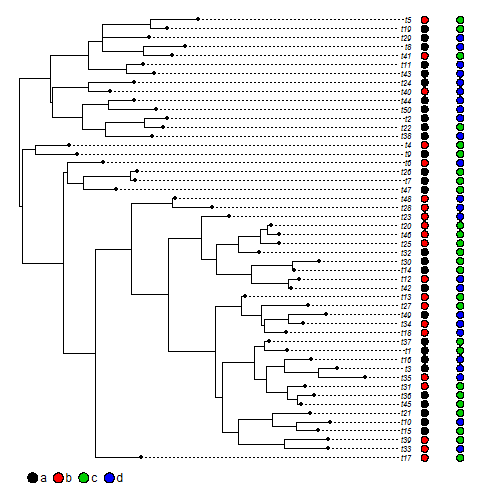``````dotTree(tree,cbind(x,y),fsize=0.7,colors=colors,x.space=0.05) ## better
``````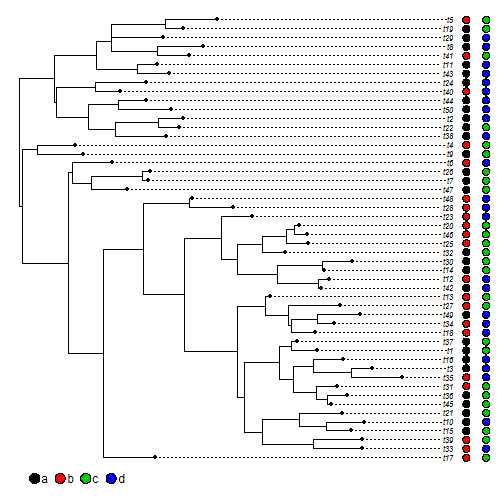The tree & data were simulated as follows:

``````tree<-rtree(n=50)
X<-fastBM(tree,nsim=20)
Q<-matrix(c(-1,1,1,-1),2,2)
rownames(Q)<-colnames(Q)<-letters[1:2]
x<-sim.history(tree,Q)\$states
rownames(Q)<-colnames(Q)<-letters[3:4]
y<-sim.history(tree,Q)\$states
``````

That's it.